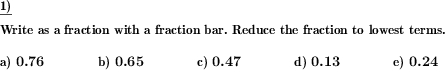Custom math worksheets at your fingertips# Details for problem "Decimal fraction to fraction bar notation"

Quickname: 1213

Elementary School, Primary School, Junior High School, Middle School, High School.

## Summary

Decimals have to be converted to fraction bar notation.

## Examples## Description

A series of decimal numbers are to be converted into fraction bar representation.

The number of decimal places can be set. The result can be requested to be reduced to lowest terms if required.

Decimal fractions can also be set as a problem, which will become improper fractions in fraction bar notation. The place before the decimal point is automatically selected accordingly. In this case, the specification can be demanded between a representation as a pure fraction or as a mixed number.

Download free printable worksheets for this math problem here. The worksheet contains the problems only, the solution sheet includes the answers. Just click on the respective link.

•Worksheet 1Solution sheet with answers
•Worksheet 2Solution sheet with answers
•Worksheet 3Solution sheet with answers

If you can not see the solution sheets for download, they may be filtered out by an ad blocker that you may have installed. If this is the case, please allow ads for this page and reload the page. The solution sheets will then reappear.

• Do these sample worksheets do not really fit?
• Do you need more math worksheets, with a different level of difficulty?
• Would you like to combine different problems on a worksheet and adjust them to your needs?
• As a teacher, you can put together your own worksheets using the automatically generated math problems provided.
With a free initial credit, you can start creating your own math worksheets in a few minutes.

You can try it for free! Register here, to create custom worksheets now!

## Customization options for this problem

Parameter
Possible values
Number of problems
1, 2, 3, 4, 5, 6, 7, 8, 9, 10
Decimal places
1, 2, 3, 4
Yes, No
Improper fractions
No, Yes, as a fraction, Yes, as a mixed number

## Similar problems

Remark
Description
Corresponding task for the opposite direction of conversion
A given fraction has to be converted to decimal notation.Deutsche Version dieser Aufgabe
These informational pages with samples describe math problems that can be combined on custom math worksheets with solutions for home and K-12 school use.
Deutsche Seiten
×